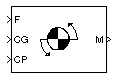# Moments about CG due to Forces

Compute moments about center of gravity due to forces applied at a point, not center of gravity

•Libraries:
Aerospace Blockset / Mass Properties

## Description

The Moments about CG due to Forces block computes moments about center of gravity due to forces that are applied at point CP, not at the center of gravity.

## Ports

### Input

expand all

Forces applied at point CP, specified as a three-element vector.

Data Types: `double`

Center of gravity, specified as three-element vector.

Data Types: `double`

Application point of forces, specified as a three-element vector.

Data Types: `double` | `bus`

### Output

expand all

Moments at the center of gravity in x-axis, y-axis and z-axis, returned as a three-element vector.

Data Types: `double`

## Version History

Introduced before R2006a